# 5. Two long straight wires are in the xy plane and parallel to the y axis....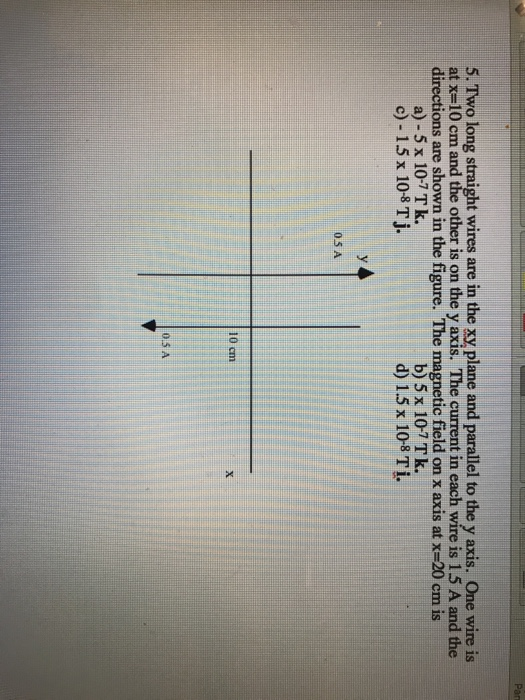5. Two long straight wires are in the xy plane and parallel to the y axis. One wire is at x=10 cmand the other is on the y axis. The current in each wire is 1.5 A and the directions are shown in the figure. The magnetic field on x axis at x=20 cm is a) - 5 x 10-7 Tk. c)-15 x 108 T j. b) 5 x 10-7Tk. d) 1.5 x 108 Ti. 05 A 10 cm 0.5 A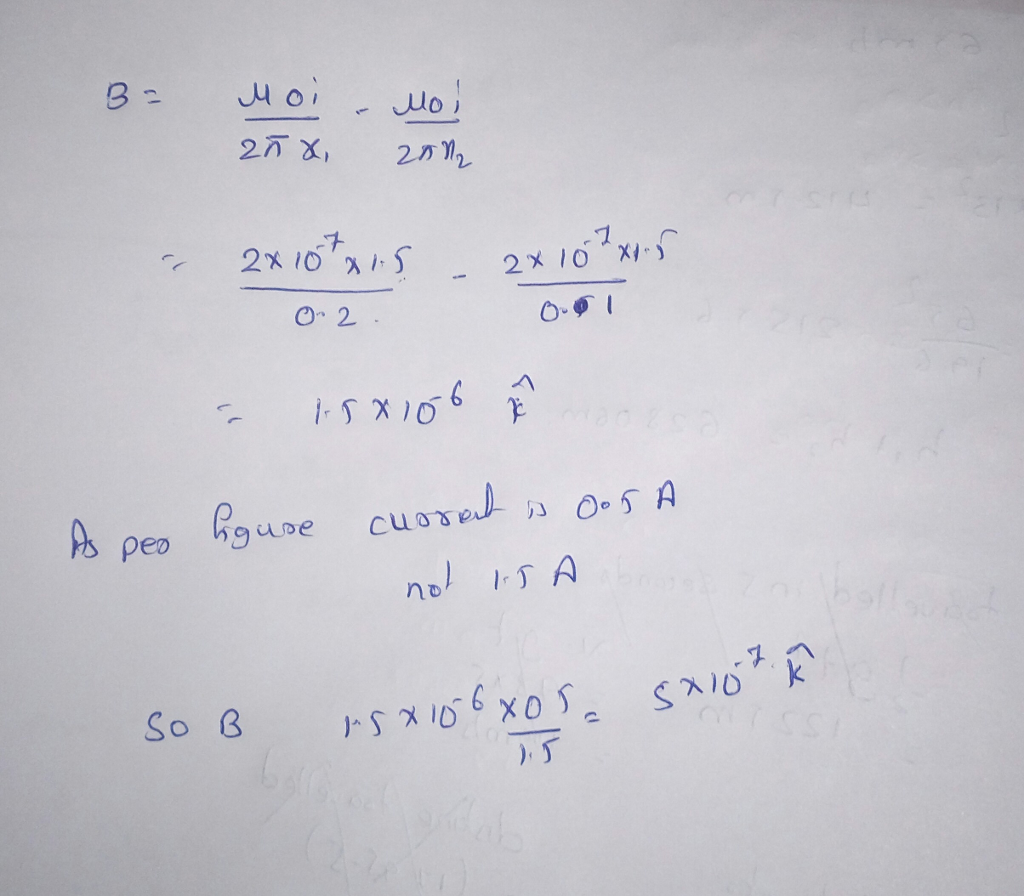#### Earn Coin

Coins can be redeemed for fabulous gifts.

Similar Homework Help Questions
• ### Magnetic Fields: Two long, straight wires in the xy plane and parallel to the x axis find the...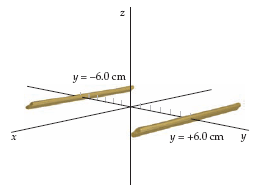The figure below shows two long, straight wires in the xy plane and parallel to the x axis. One wire isat y = −6.0 cm and the other wire is at y = +6.0 cm. The current in each wire is 34 A. Ifthe currents are both in the −x direction, find the magnetic field at the following points on the y axis.(a) y = −1.0 cm________________ µT (b) y = 0_______0_____ µT (c) y = +1.0 cm________________ µT (d)...

• ### Two long straight wires are parallel to the y axis, with one at x = 0...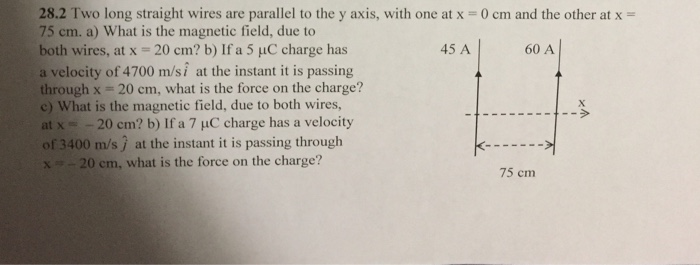Two long straight wires are parallel to the y axis, with one at x = 0 cm and the other at x = 75 cm. What is the magnetic field, due to both wires, at x = 20 cm? If a 5 mu C charge has a velocity of 4700 m/si at the instant it is passing through x = 20 cm, what is the force on the charge? What is the magnetic field, due to both wires, at x...

• ### Two infinitely long parallel wires carry current in -k direction the wire that intersect xy plane...

Two infinitely long parallel wires carry current in -k direction the wire that intersect xy plane on the x-axis at x= -3m carries 20A of current. The second wire intersects xy plan at x=+3m and carries 40A. Find the y component of the total magnetic field on y axis at y =4 Find the force per meter

• ### Question 20 (7 points) Two long straight wires carry currents perpendicular to the xy-plane. One wire...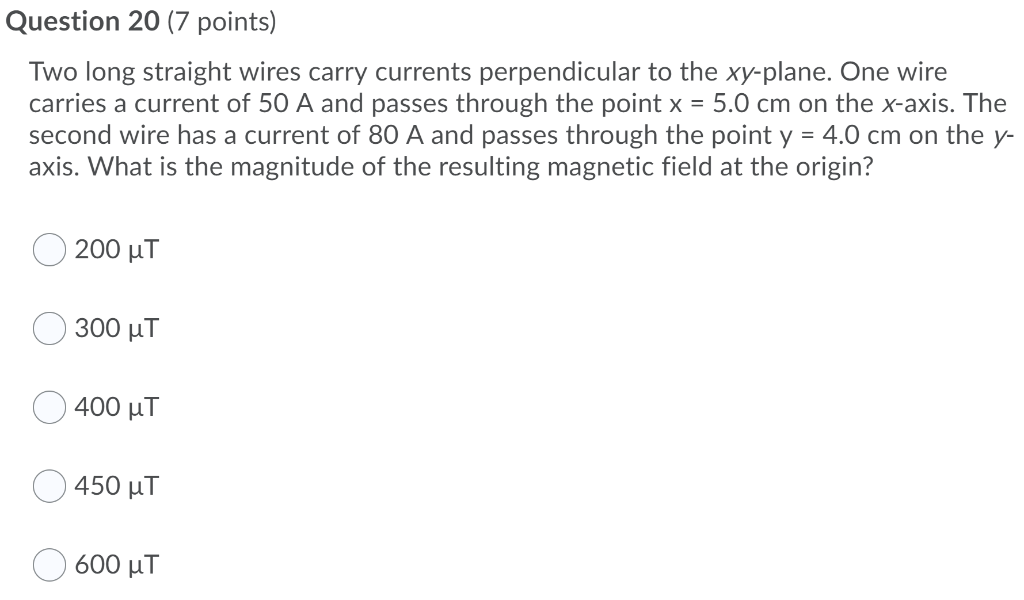Question 20 (7 points) Two long straight wires carry currents perpendicular to the xy-plane. One wire carries a current of 50 A and passes through the point x = 5.0 cm on the x-axis. The second wire has a current of 80 A and passes through the point y = 4.0 cm on the y- axis. What is the magnitude of the resulting magnetic field at the origin? 200 ut 300 uT 400 uT 450 uT 600 uT

• ### Magnetic Field in parallel wires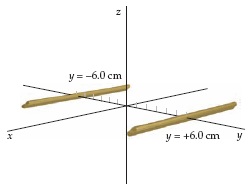The figure below shows two long, straight wires in the xy plane and parallel to the x axis. One wire isat y = -6.0 cm and the other wire is at y = +6.0 cm. The current in each wire is 19 A.(a) Find the magnetic field on the z axis at z = +8.6 cm if the currents are both in the-x direction.µT(b) Find the magnetic field on the z axis at z = +8.6 cm if the current...

• ### Two infinitely long, straight parallel wires perpendicular to the plane of the page carry currents of...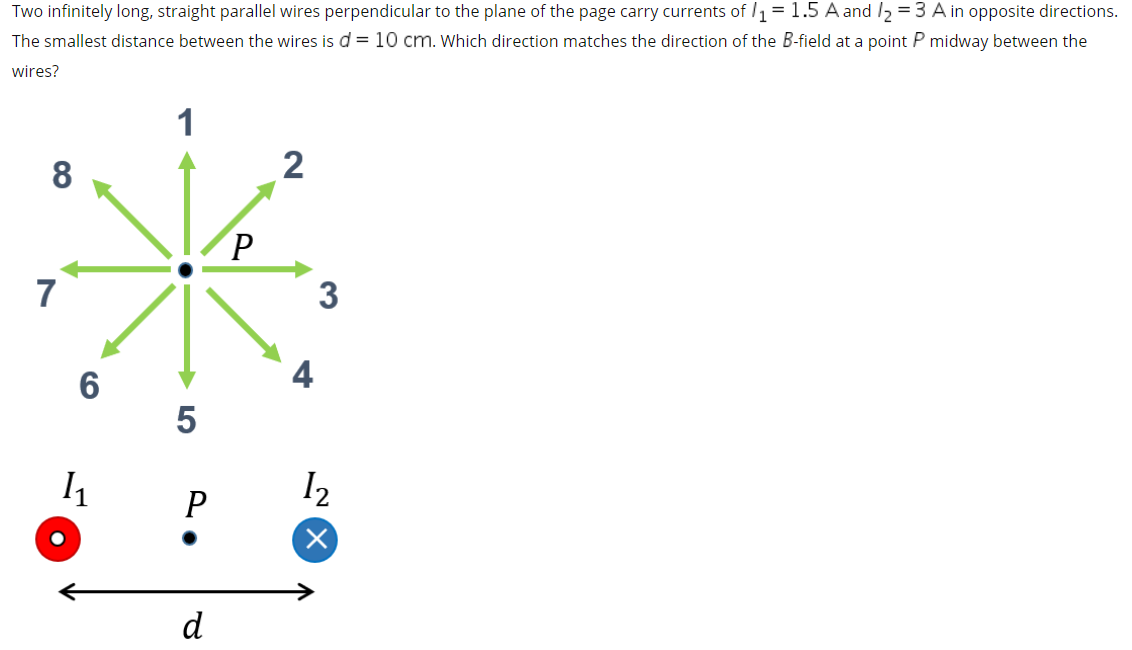Two infinitely long, straight parallel wires perpendicular to the plane of the page carry currents of l1 = 1.5 A and I2 = 3 A in opposite directions. The smallest distance between the wires is d = 10 cm. Which direction matches the direction of the B-field at a point P midway between the wires?

• ### Two Wires Points: 1 Consider two parallel conducting wires along the direction of the Z axis...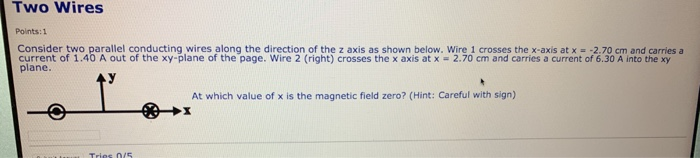Two Wires Points: 1 Consider two parallel conducting wires along the direction of the Z axis as shown below. Wire 1 crosses the x-axis at x = -2.70 cm and carries a current of 1.40 A out of the xy-plane of the page. Wire 2 (right) crosses the x axis at x = 2.70 cm and carries a current of 6.30 A into the xy plane. At which value of x is the magnetic field zero? (Hint: Careful with sign)...

• ### Three long straight wires are parallel to the z-axis carrying equal currents of 10 A in...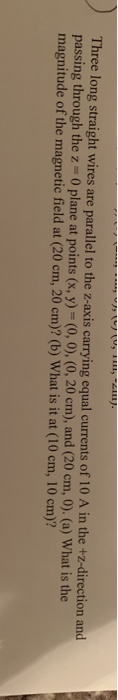Three long straight wires are parallel to the z-axis carrying equal currents of 10 A in the +z-direction and passing through the z 0 plane at points (x, y) (0, 0), (0, 20 cm), and (20 cm, 0). (a) What is the magnitude of the magnetic field at (20 cm, 20 cm)? (b) What is it at (10 cm, 10 cm)?

• ### 1. Two long wires are parallel to one another and the y- axis. The wires are...

1. Two long wires are parallel to one another and the y- axis. The wires are separated by a distance of 15 cm apart. The wire on the left has a current of 2.5 A in the upward direction and the wire on the right has a current of 1.5 A in the upward direction. What is the magnetic field half way between the wires. A. 2.7 µT inward B. 10.7 µT outward C. 10.7 µT inward D. 2.7 µT...

• ### Two long (infinite) wires are placed in the x-y plane parallel to one another. One is...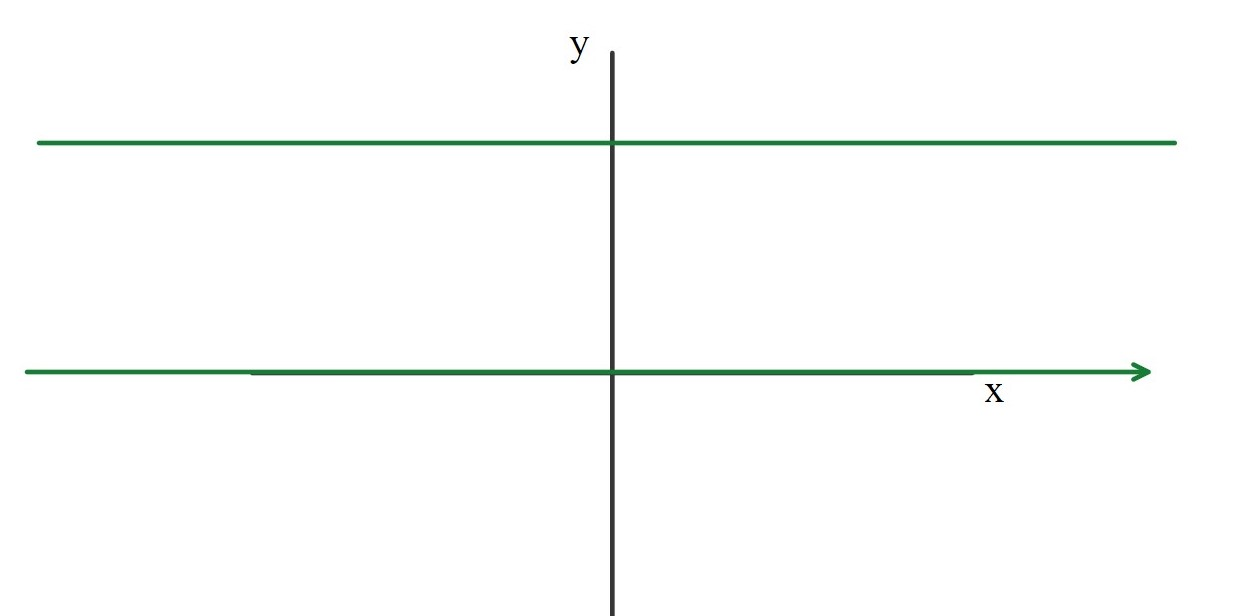Two long (infinite) wires are placed in the x-y plane parallel to one another. One is placed on the x axis and the current in that wire is 14 amps running in the +x direction. The other wire is placed at y = +2.6 meters (2.6 meters "above" the first wire, in the x-y plane). The second wire has a force per unit length of 24 x 10-7 N/m in the -y direction. Where, on the y axis, is the...